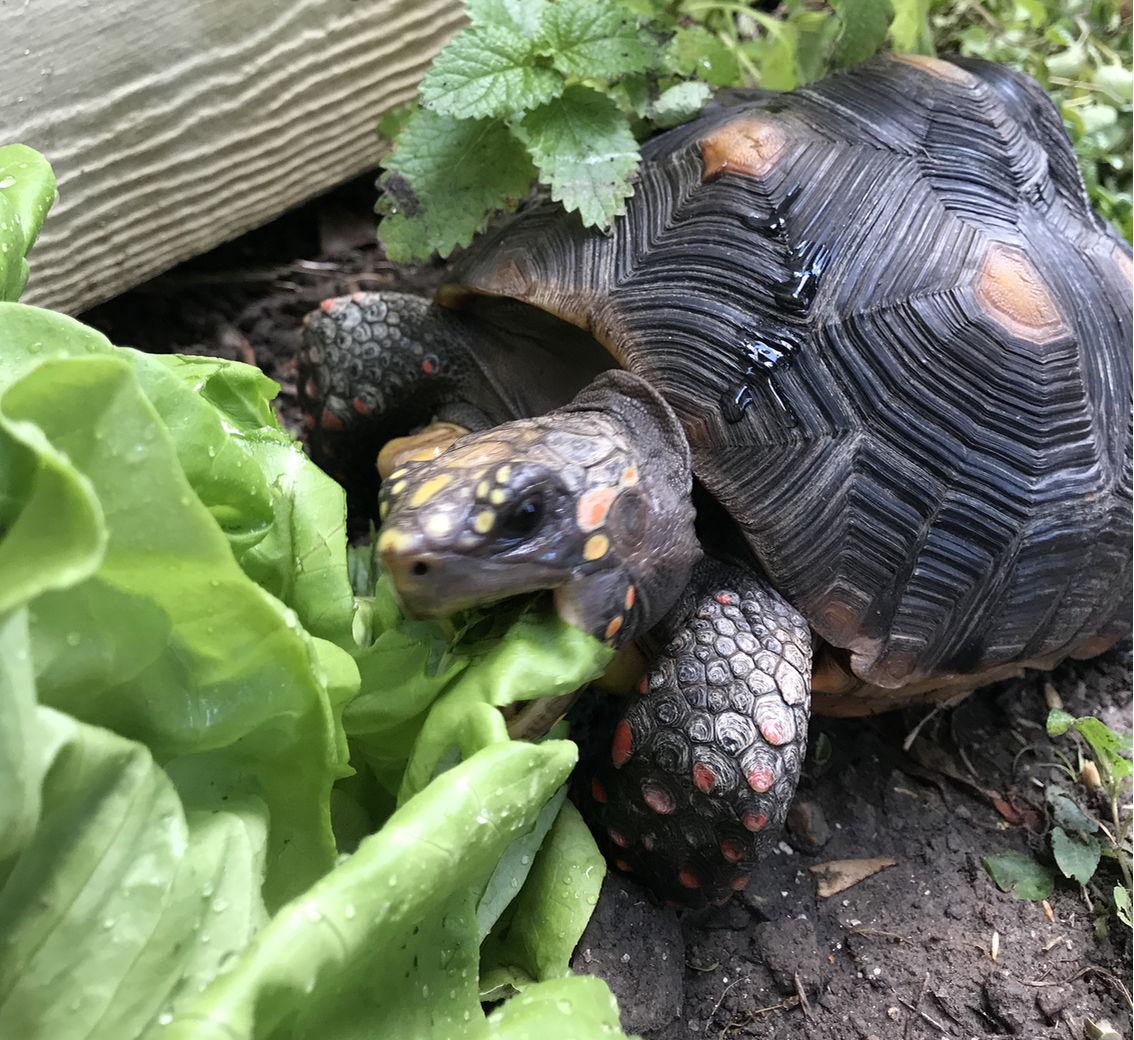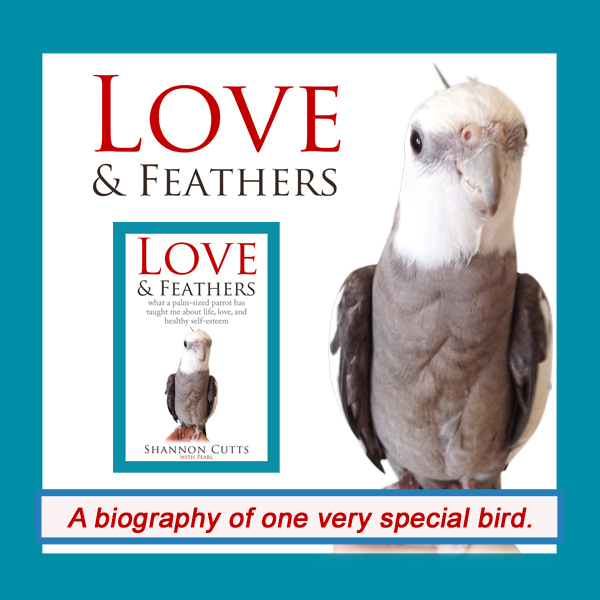# July 2019

 table div table+table+table div table{width:100%;padding:0}table div table+table+table div table img{width:96.23%;padding:0;float:none}table div table+table+table div table td{width:100%;padding:0 1.88% 18px}/* styles *//* styles */ Receiving this email as a forward? Sign up to keep following our little flock's adventures!
 table div table+table+table+table+table+table div table{width:100%;padding:0}table div table+table+table+table+table+table div table img{width:96.23%;padding:0;float:none}table div table+table+table+table+table+table div table td{width:100%;padding:0 1.88% 18px}/* styles */## Faith & Confidence: Could They Be One & the Same?Sometimes I have to resort to "redirection" to give the freshly scattered seeds time to sprout and grow....in this case, by "planting" a living lettuce directly in the, well, damage path.

Over the last month or so, I've been working my way through a wonderful book called "Your Heart's Desire" by author and intuitive teacher Sonia Choquette.

This book, first published back in 1995, is precisely the book I need now in 2019. If I had read it then, when I would have just been graduating college and launching into a new and extraordinarily ill-chosen career in the oil industry, it probably would have broken my heart.

But today, it lifts my heart and gives me so much more than hope. It gives me practical steps I can take to tweak and adjust and ponder and reframe and comprehend and heal and move ahead with confidence.

Or, as Sonia prefers to call it, with faith.

In the book, she writes:

One of my favorite definitions of faith is "confidence in the future based on what you have done in the past."

Can I just tell you how my mind broke out into its own peculiar version of the happy dance when it read this?

For so many years, decades really, my mind has been insisting that a reliance on "faith" is my weenie heart's weak attempt to get out of doing the brass tacks hard work of course setting, and, of course, course correction.

My heart, on the contrary, has had its firm little feet planted deep down in some particularly sticky mud, refusing to budge an inch from its insistence on faith as a necessary part of any transformative equation no matter how much my mind heckled.

Now, the two of them are not quite sure what to do with each other. I can almost feel the inner staring contest as the hostility drains away and curiosity takes its place.

In other words, it would appear that one says "confidence" and the other says "faith." But beneath surface semantics, they quite possibly have been referring to the same thing all along.

I love this. My whole life, starting back way before I had any idea what I was talking about when I would say this, I have absolutely insisted that the only way to create a life worth living is to take care of the whole person - the entire entity - the complete me.

Body, mind, heart, spirit - like four wheels of a car, they really really really do all need to be pointing in the same direction, fully aired up, aligned, rotated, robust with tread and all that good stuff before the car is going to move reliably and safely forward.

Or backward, or left, or right, or whichever direction the group consensus elects to take us at any given moment.

Saying this is one thing. Doing it, of course, is something else entirely.

For nearly all of my life to date, my four tires have been steadfastly intent on doing their own thing. Individualists to their core, my brain loves logic, reason, analysis. My heart enjoys floating around while surfing the emotional airwaves. My body, well, that is a whole other topic that has been well-covered in various blogs, articles and books over the years.

And my spirit - all I can say here is, I'm lucky the other three didn't ever manage to agree for long enough to gang up on it and send it where the sun doesn't shine.

Today, however, we are all seriously intrigued with what it might be like to actually work together. What if we pooled our talents, our strengths, our gifts? What would life feel like if, instead of continual inner squabbles, we could each count on real moments of extended daily peace?

Could we perhaps co-create a life and a lifestyle where both confidence and faith matter, are valued, are available and used each and every day?

In her book, Sonia gives an example of how confidence/faith fits in to a life of sincere striving and daily significant self-effort towards personal life goals.

She uses the analogy of a farmer planting seeds. I find this particularly relevant. Our little flock lives in Texas, a state where everything is not only bigger but also much hotter. As summer rolls out in earnest and flattens nearly every living thing it comes in contact with, I have been working hard on a daily basis to populate my two turtles' outdoor play area with edible greens they can forage for in the way they would out in the wild.

But every single dang time I head out to sprinkle the grass seeds, guess who comes along right behind me and snarfs them up? (If you guessed Malti, my young and perpetually ravenous tortoise, you win a gold star).

Sonia talks about how the farmer can do all that hard work, scattering the seeds, tilling the soil, watering them, weeding them, fertilizing them, doing all the manual labor type things it takes to make sure each seed has its best chance to unfurl, sprout, shoot skyward, strengthen and finally mature.

Yet, she says, ultimately the farmer can't make the seeds grow. That is someone else's job, and even with all our science and knowledge and study of genomes and DNA and optimal growing conditions, we still can't completely or with full confidence (faith?) explain precisely what activates a seed to do its thing.

So this analogy makes perfect sense to both my brain and my heart. My body understands it too in its own non-verbal yet deeply reverent way - after all, after a long and arduous journey out of sickness spanning multiple decades, all its hard work finally paid off, but not a one of us could point out the single action that finally spelled success.

So now, when my heart speaks of faith, prays for guidance, activates intuition, meditates, contemplates, my brain doesn't argue with it. Instead, my mind uses its new "heart to brain" translation dictionary, understanding at last that when my heart says "faith," what it really means is "confidence."

And they all lived happily ever after.

with great love and respect,

Shannon

 table div table+table+table+table+table+table+table+table+table div table{width:100%;padding:0}table div table+table+table+table+table+table+table+table+table div table img{width:96.23%;padding:0;float:none}table div table+table+table+table+table+table+table+table+table div table td{width:100%;padding:0 1.88% 18px}/* styles *//* styles */ Have you had to work with yourself to find alternate expressions for cherished concepts or words when any aspect of you rebels, disbelieves, balks in fear or rage? What does your mind think about using the word "confidence" when your heart speaks of "faith?" I'd love to hear your ideas and insights!
 table div table+table+table+table+table+table+table+table+table+table+table div table{width:100%;padding:0}table div table+table+table+table+table+table+table+table+table+table+table div table img{width:96.23%;padding:0;float:none}table div table+table+table+table+table+table+table+table+table+table+table div table td{width:100%;padding:0 1.88% 18px}/* styles */# Treat Yourself to Tasty Waffles & More!

Enjoy 70 of Pearl's favorite "people food" recipes, made with love by his personal Small Chef, his grandma.

Crispy waffles, fluffy waffles, crepes, bread, scones, pasta, pizza, potatoes, sweets, even gluten-free treats the whole flock can enjoy....it's all in here!

 table.module-12{width:75.47%;padding:0}table div table+table+table+table+table+table+table+table+table+table+table+table+table div table{width:75.47%;float:none;margin-left:auto;margin-right:auto;padding:0}table div table+table+table+table+table+table+table+table+table+table+table+table+table div table a{border:0 none;text-decoration:none}table div table+table+table+table+table+table+table+table+table+table+table+table+table div table img{width:100%!important;border:0 none;text-decoration:none}table div table+table+table+table+table+table+table+table+table+table+table+table+table div table td{width:100%;padding:0}/* styles */
 table div table+table+table+table+table+table+table+table+table+table+table+table+table+table+table div table{width:100%;padding:0}table div table+table+table+table+table+table+table+table+table+table+table+table+table+table+table div table img{width:96.23%;padding:0;float:none}table div table+table+table+table+table+table+table+table+table+table+table+table+table+table+table div table td{width:100%;padding:0 1.88% 18px}/* styles */# Get your copy of Love & Feathers!

I have always loved all animals, but birds have become my heart. And that is why I like your book so much. You touch the bird in me.
–Ellen Cook, DVM

Shannon, I just finished your book. It’s the best book I’ve ever read. I absolutely loved it. I’ve had books that I quit reading from the library because I get bored so easily. I could not put it down. I read every word. Thank you so much! You really are a special bird mom! And Tort mom as well!
–Marta CrabtreeClick on the image of Pearl to order your signed copy!Pearl will even beak-o-graph it for you!
 table div table+table+table+table+table+table+table+table+table+table+table+table+table+table+table+table+table+table+table+table div table{width:100%;padding:0}table div table+table+table+table+table+table+table+table+table+table+table+table+table+table+table+table+table+table+table+table div table img{width:96.23%;padding:0;float:none}table div table+table+table+table+table+table+table+table+table+table+table+table+table+table+table+table+table+table+table+table div table td{width:100%;padding:0 1.88% 18px}/* styles */# Summer is for chowing on green growing things!

 table.module-21{width:75.47%;padding:0}table div table+table+table+table+table+table+table+table+table+table+table+table+table+table+table+table+table+table+table+table+table+table div table{width:75.47%;float:none;margin-left:auto;margin-right:auto;padding:0}table div table+table+table+table+table+table+table+table+table+table+table+table+table+table+table+table+table+table+table+table+table+table div table a{border:0 none;text-decoration:none}table div table+table+table+table+table+table+table+table+table+table+table+table+table+table+table+table+table+table+table+table+table+table div table img{width:100%!important;border:0 none;text-decoration:none}table div table+table+table+table+table+table+table+table+table+table+table+table+table+table+table+table+table+table+table+table+table+table div table td{width:100%;padding:0}/* styles */
 table div table+table+table+table+table+table+table+table+table+table+table+table+table+table+table+table+table+table+table+table+table+table+table+table div table{width:100%;padding:0}table div table+table+table+table+table+table+table+table+table+table+table+table+table+table+table+table+table+table+table+table+table+table+table+table div table img{width:96.23%;padding:0;float:none}table div table+table+table+table+table+table+table+table+table+table+table+table+table+table+table+table+table+table+table+table+table+table+table+table div table td{width:100%;padding:0 1.88% 18px}/* styles */# Bruce makes "hitting the hay" look extra-good.

 table.module-25{width:75.47%;padding:0}table div table+table+table+table+table+table+table+table+table+table+table+table+table+table+table+table+table+table+table+table+table+table+table+table+table+table div table{width:75.47%;float:none;margin-left:auto;margin-right:auto;padding:0}table div table+table+table+table+table+table+table+table+table+table+table+table+table+table+table+table+table+table+table+table+table+table+table+table+table+table div table a{border:0 none;text-decoration:none}table div table+table+table+table+table+table+table+table+table+table+table+table+table+table+table+table+table+table+table+table+table+table+table+table+table+table div table img{width:100%!important;border:0 none;text-decoration:none}table div table+table+table+table+table+table+table+table+table+table+table+table+table+table+table+table+table+table+table+table+table+table+table+table+table+table div table td{width:100%;padding:0}/* styles */
 table div table+table+table+table+table+table+table+table+table+table+table+table+table+table+table+table+table+table+table+table+table+table+table+table+table+table+table+table div table{width:100%;padding:0}table div table+table+table+table+table+table+table+table+table+table+table+table+table+table+table+table+table+table+table+table+table+table+table+table+table+table+table+table div table img{width:96.23%;padding:0;float:none}table div table+table+table+table+table+table+table+table+table+table+table+table+table+table+table+table+table+table+table+table+table+table+table+table+table+table+table+table div table td{width:100%;padding:0 1.88% 18px}/* styles */## Meet Pearl, Malti, Bruce & Me

 table div table+table+table+table+table+table+table+table+table+table+table+table+table+table+table+table+table+table+table+table+table+table+table+table+table+table+table+table+table+table div table,table.module-29{width:34.34%;float:left;padding:0}table div table+table+table+table+table+table+table+table+table+table+table+table+table+table+table+table+table+table+table+table+table+table+table+table+table+table+table+table+table+table div table a{border:0 none;text-decoration:none}table div table+table+table+table+table+table+table+table+table+table+table+table+table+table+table+table+table+table+table+table+table+table+table+table+table+table+table+table+table+table div table img{width:100%!important;border:0 none;text-decoration:none}table div table+table+table+table+table+table+table+table+table+table+table+table+table+table+table+table+table+table+table+table+table+table+table+table+table+table+table+table+table+table div table td{width:100%;padding:0 20px 20px 0}/* styles */ Hi! It is so good to meet you here each month! Pearl, Malti, Bruce and their mommy are delighted you are in our extended flock! We are so excited about our newest book, "Waffles & More: A Love & Feathers Recipe Book, which features 70 "people food" recipes the whole flock can enjoy! Have you treated yourself yet to a copy of "Love & Feathers: what a palm-sized parrot has taught me about life, love, and healthy self-esteem?" Get ready to smile, laugh, fall in love with your own pets all over again! ==> To connect with me: www.shannoncutts.com ==> To connect with Pearl, Malti & Bruce: www.loveandfeathersandshells.com
 table div table+table+table+table+table+table+table+table+table+table+table+table+table+table+table+table+table+table+table+table+table+table+table+table+table+table+table+table+table+table+table div table{width:100%;padding:0}table div table+table+table+table+table+table+table+table+table+table+table+table+table+table+table+table+table+table+table+table+table+table+table+table+table+table+table+table+table+table+table div table img{width:96.23%;padding:0;float:none}table div table+table+table+table+table+table+table+table+table+table+table+table+table+table+table+table+table+table+table+table+table+table+table+table+table+table+table+table+table+table+table div table td{width:100%;padding:0 1.88% 18px}/* styles */table div table+table+table+table+table+table+table+table+table+table+table+table+table+table+table+table+table+table+table+table+table+table+table+table+table+table+table+table+table+table+table+table div table td,table.module-31{width:100%;padding:0}table div table+table+table+table+table+table+table+table+table+table+table+table+table+table+table+table+table+table+table+table+table+table+table+table+table+table+table+table+table+table+table+table div table{width:100%;float:none;margin-left:auto;margin-right:auto;padding:0}table div table+table+table+table+table+table+table+table+table+table+table+table+table+table+table+table+table+table+table+table+table+table+table+table+table+table+table+table+table+table+table+table div table a{border:0 none;text-decoration:none}table div table+table+table+table+table+table+table+table+table+table+table+table+table+table+table+table+table+table+table+table+table+table+table+table+table+table+table+table+table+table+table+table div table img{width:100%!important;border:0 none;text-decoration:none}/* styles */
 table.module-32{width:74.15%;padding:0}table div table+table+table+table+table+table+table+table+table+table+table+table+table+table+table+table+table+table+table+table+table+table+table+table+table+table+table+table+table+table+table+table+table div table{width:74.15%;float:none;margin-left:auto;margin-right:auto;padding:0}table div table+table+table+table+table+table+table+table+table+table+table+table+table+table+table+table+table+table+table+table+table+table+table+table+table+table+table+table+table+table+table+table+table div table a{border:0 none;text-decoration:none}table div table+table+table+table+table+table+table+table+table+table+table+table+table+table+table+table+table+table+table+table+table+table+table+table+table+table+table+table+table+table+table+table+table div table img{width:100%!important;border:0 none;text-decoration:none}table div table+table+table+table+table+table+table+table+table+table+table+table+table+table+table+table+table+table+table+table+table+table+table+table+table+table+table+table+table+table+table+table+table div table td{width:100%;padding:0}/* styles */
 table div table+table+table+table+table+table+table+table+table+table+table+table+table+table+table+table+table+table+table+table+table+table+table+table+table+table+table+table+table+table+table+table+table+table div table{width:100%;padding:0}table div table+table+table+table+table+table+table+table+table+table+table+table+table+table+table+table+table+table+table+table+table+table+table+table+table+table+table+table+table+table+table+table+table+table div table img{width:96.23%;padding:0;float:none}table div table+table+table+table+table+table+table+table+table+table+table+table+table+table+table+table+table+table+table+table+table+table+table+table+table+table+table+table+table+table+table+table+table+table div table td{width:100%;padding:0 1.88% 18px}/* styles */## Reprints & Reposts

REPRINTS: Would you like to use any portion of this material in your newsletter, blog or website? You may do so as long as you also include this copyright information: